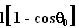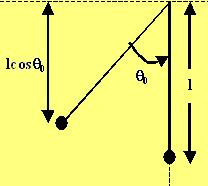## Saturday, October 20, 2007

### Irodov Problem 1.86Let the initial angle where the ball was left off from be. The force acting on the ball in the tangential direction of motion will be(as shown in the figure). The acceleration in the tangential direction will thus be. There will be no radial component to the acceleration since the ball is stationary at this position.

The velocity of the ball at the lowest position can be determined by using conservation of energy. As shown in the figure, the height the ball would have fallen is. Thus,At the lowest point the only acceleration the ball is subjected to is the centripetal acceleration acting radially upwards and is equal to.

If both these accelerations are to be equal then,mukul said...

thank u kant sir 4 this great work

hattts off to you

my id
masmuku@uymail.com